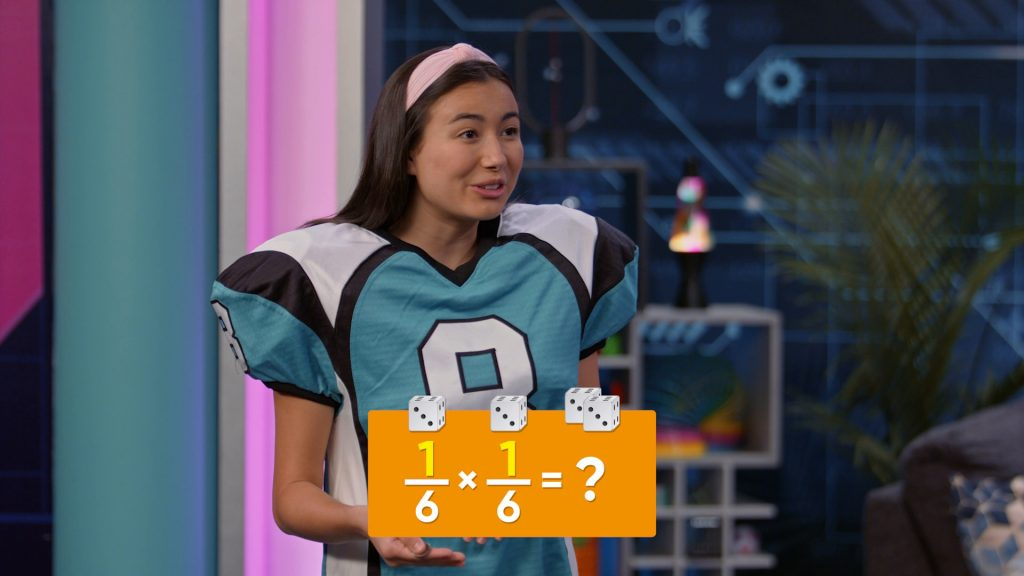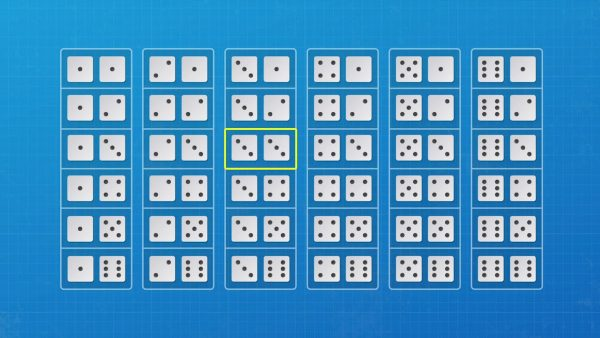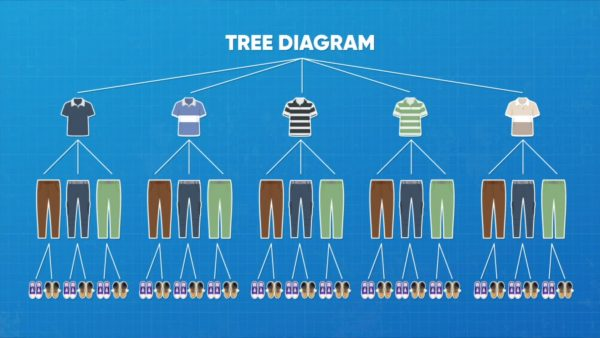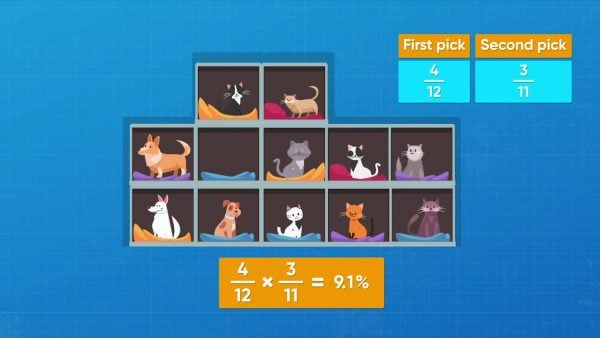Probability: Compound Events | Math for kids Grade 6, 7, & 8
1%
It was processed successfully!WHAT ARE COMPOUND EVENTS ?

In probability, compound events are two or more events that happen together. For example, two coins are flipped or one coin is flipped and then flipped a second time.

To better understand compound events…

WHAT ARE COMPOUND EVENTS ?. In probability, compound events are two or more events that happen together. For example, two coins are flipped or one coin is flipped and then flipped a second time.  To better understand compound events…

## LET’S BREAK IT DOWN!

### Calculate the probability of flipping a coin and getting heads twice.Let’s say you want to flip a coin twice and calculate the chance of getting heads twice. This is a compound event, since it is two events happening together: two coin flips. First, calculate the probability of getting heads on one coin toss. There are two possible outcomes, heads or tails, and you are looking for one of those outcomes: heads. The probability of getting heads on one coin toss is 12. To find the compound probability, you can list all of the possible outcomes: [H, H], [H, T], [T, H], [T, T]. There are 4 possible outcomes. Only one of the outcomes is 2 heads. The probability of getting heads twice is 14 or 25%. Try this one yourself. If you toss two coins, what is the probability of getting 1 head and 1 tail?

Calculate the probability of flipping a coin and getting heads twice. Let’s say you want to flip a coin twice and calculate the chance of getting heads twice. This is a compound event, since it is two events happening together: two coin flips. First, calculate the probability of getting heads on one coin toss. There are two possible outcomes, heads or tails, and you are looking for one of those outcomes: heads. The probability of getting heads on one coin toss is 12. To find the compound probability, you can list all of the possible outcomes: [H, H], [H, T], [T, H], [T, T]. There are 4 possible outcomes. Only one of the outcomes is 2 heads. The probability of getting heads twice is 14 or 25%. Try this one yourself. If you toss two coins, what is the probability of getting 1 head and 1 tail?

### Find the probability of rolling 3 on both of two number cubes.Let’s say you want to toss two number cubes and find the possibility of getting a 3 on both of them. Each number cube has the numbers 1 through 6. The probability of rolling a 3 on one number cube is 16. What is the probability of rolling a 3 on both number cubes? If you roll a 1 on the first cube, you can roll any of the 6 numbers on the second roll. The same pattern continues if you roll a 2 on the first cube. You can make a table, like the image you see on the right, to show all of the possible outcomes. There are 36 possible outcomes. The outcome 3, 3 is only in the table once. So the probability of rolling two 3’s on the number cubes is 136. The probability of getting each event on its own is 16. If you multiply 16 × 16 you also get 136. For compound events like this, you can multiply the probabilities of the two events to find the probability of the compound event. Try this one yourself. What is the probability of tossing a 4 and then a 6 on a number cube?

Find the probability of rolling 3 on both of two number cubes. Let’s say you want to toss two number cubes and find the possibility of getting a 3 on both of them. Each number cube has the numbers 1 through 6. The probability of rolling a 3 on one number cube is 16. What is the probability of rolling a 3 on both number cubes? If you roll a 1 on the first cube, you can roll any of the 6 numbers on the second roll. The same pattern continues if you roll a 2 on the first cube. You can make a table, like the image you see on the right, to show all of the possible outcomes. There are 36 possible outcomes. The outcome 3, 3 is only in the table once. So the probability of rolling two 3’s on the number cubes is 136. The probability of getting each event on its own is 16. If you multiply 16 × 16 you also get 136. For compound events like this, you can multiply the probabilities of the two events to find the probability of the compound event. Try this one yourself. What is the probability of tossing a 4 and then a 6 on a number cube?

### Calculate the probability of picking a certain outfit.Let’s say you have 5 different shirts, 3 different pairs of pants, and 2 different pairs of shoes. You want to find the probability of picking the blue shirt, the blue pants, and the blue shoes if you choose at random. Make a tree diagram to show all of the possible outcomes. You start with a row that contains a list of the five possible shirts. Now you connect each different shirt with 3 different pants by drawing 3 branches from each shirt. And then you branch out again with 2 branches from each pants for the two different shoe possibilities. You can see from the tree diagram that there are 30 possible outcomes and only one of them is {blue shirt, blue pants, blue shoes}. So the probability of choosing the blue shirt, the blue pants, and the blue shoes is [ggfrac]1/30[/ggfrac]. You could also multiply [ggfrac]1/5[/ggfrac] × [ggfrac]1/3[/ggfrac] × [ggfrac]1/2[/ggfrac] to find the same probability of[ggfrac]1/30[/ggfrac]. Try this one yourself. Your friend has 3 shirts {blue, red, yellow}, 2 pairs of pants {brown, gray}, and 2 pairs of shoes {white, black}. What is the probability of randomly choosing an outfit and getting the red shirt, the gray pants, and the black shoes?

Calculate the probability of picking a certain outfit. Let’s say you have 5 different shirts, 3 different pairs of pants, and 2 different pairs of shoes. You want to find the probability of picking the blue shirt, the blue pants, and the blue shoes if you choose at random. Make a tree diagram to show all of the possible outcomes. You start with a row that contains a list of the five possible shirts. Now you connect each different shirt with 3 different pants by drawing 3 branches from each shirt. And then you branch out again with 2 branches from each pants for the two different shoe possibilities. You can see from the tree diagram that there are 30 possible outcomes and only one of them is {blue shirt, blue pants, blue shoes}. So the probability of choosing the blue shirt, the blue pants, and the blue shoes is [ggfrac]1/30[/ggfrac]. You could also multiply [ggfrac]1/5[/ggfrac] × [ggfrac]1/3[/ggfrac] × [ggfrac]1/2[/ggfrac] to find the same probability of[ggfrac]1/30[/ggfrac]. Try this one yourself. Your friend has 3 shirts {blue, red, yellow}, 2 pairs of pants {brown, gray}, and 2 pairs of shoes {white, black}. What is the probability of randomly choosing an outfit and getting the red shirt, the gray pants, and the black shoes?

### Calculate the probability of picking two dogs from a pet store.Let’s say Amari and Emily are at a pet store and they each want to get a dog. The pet store has 4 dogs and 8 cats. Find the probability of both friends picking a dog if they choose a pet at random. These two events are not independent of each other. The probability of the second event is dependent on what happens in the first pick. In the first event, there are 12 total pets and 4 possible ways to get a dog. If Amari picks first, the probability that she picks a dog it 4 out of 12, or [ggfrac] 4/12[/ggfrac]For Emily, there are now 11 pets and 3 possible ways to pick a dog. The probability that Emily picks a dog is 3 out of 11, or [ggfrac] 3/11[/ggfrac]. You can multiply to find the probability of the friends picking two dogs. [ggfrac]4/12 [/ggfrac] × [ggfrac]3/11 [/ggfrac] = [ggfrac] 12/132[/ggfrac], or 0.9.9.9... You can state the probability as a percent and round it to the nearest tenth of a percent. The probability of Amari and Emily both getting dogs is 9.1%. Try this one yourself. In Amari and Emily’s situation, what is the probability of both friends getting cats instead of dogs?

Calculate the probability of picking two dogs from a pet store. Let’s say Amari and Emily are at a pet store and they each want to get a dog. The pet store has 4 dogs and 8 cats. Find the probability of both friends picking a dog if they choose a pet at random. These two events are not independent of each other. The probability of the second event is dependent on what happens in the first pick. In the first event, there are 12 total pets and 4 possible ways to get a dog. If Amari picks first, the probability that she picks a dog it 4 out of 12, or [ggfrac] 4/12[/ggfrac]For Emily, there are now 11 pets and 3 possible ways to pick a dog. The probability that Emily picks a dog is 3 out of 11, or [ggfrac] 3/11[/ggfrac]. You can multiply to find the probability of the friends picking two dogs. [ggfrac]4/12 [/ggfrac] × [ggfrac]3/11 [/ggfrac] = [ggfrac] 12/132[/ggfrac], or 0.9.9.9... You can state the probability as a percent and round it to the nearest tenth of a percent. The probability of Amari and Emily both getting dogs is 9.1%. Try this one yourself. In Amari and Emily’s situation, what is the probability of both friends getting cats instead of dogs?

## PROBABILITY: COMPOUND EVENTS VOCABULARY

Probability
The likelihood that an event occurs. Probability is expressed using numbers between 0 and 1.
Event
A set of possible outcomes that result from a particular experiment or situation.
Compound Events
Two or more events happening together.
Dependent Events
Events that depend on each other, where one event has an effect on another event.
Independent Events
Events that don’t depend on each other, where one event does not have an effect on other events.
Impossible Event
An event that has a probability of 0 of occurring.
An event that has a probability of 1 of occurring.
Any potential result in an experiment. For example, when you flip a coin there are two outcomes: {heads, tails}.
A diagram that has branches and shows all the possible outcomes of an event.

## PROBABILITY: COMPOUND EVENTS DISCUSSION QUESTIONS

### What are the methods you can use to find the probability of an independent compound event?

Sample answers: make a table, draw a tree diagram, find the probabilities as single events and then multiply them to find the probability of the compound event

### When you calculate the probability of dependent events, what do you need to do after you have completed the first event?

Once you have completed the first event, you need to see how the number of possible outcomes has changed for the second event. You also need to see if the number of favorable outcomes has changed.

### What is the probability of tossing three coins and having them all come up heads?

Make a table or list to show the outcomes:

H H H

H T H

H H T

H T T

T T T

T H T

T T H

T H H

Only one of these 8 outcomes is H H H, so the probability of getting 3 heads is 18.

or

The probability of getting heads on a toss of a coin is 12. So the probability of getting 3 heads when tossing 3 coins is [ggfrac] 1/2[/ggfrac] × [ggfrac] 1/2[/ggfrac] × [ggfrac] 1/2[/ggfrac], or [ggfrac] 1/8[/ggfrac].

### Ms. Lee is having a dinner party. These are the choices for the menu:Main dish: steak, chickenVegetable: corn, green beans, broccoliDessert: lemon tart, strawberry ice creamShe decides to choose something from each list randomly to create the menu. Create a tree diagram that shows the probability of having chicken, broccoli, and strawberry ice cream at her dinner party.

The tree diagram shows the choices of steak and chicken in the top row. Three branches from each main dishes show corn, green beans, and broccoli. Two branches from each vegetable show lemon tart and strawberry ice cream. The tree diagram shows that there are 12 different possibilities for the menu. Only one of those possibilities is chicken, broccoli, and strawberry ice cream. So the probability of having chicken, broccoli, and strawberry ice cream at her dinner party is [ggfrac]1/12[/ggfrac].

### A bag of marbles contains 6 blue marbles and 4 yellow marbles. Suppose you pick a marble out of the bag without looking and don’t put it back in the bag. Then you pick another marble out of the bag. What is the probability that you get a blue marble on the first pick and a yellow marble on the second pick?

There are 6 blue marbles, 4 yellow marbles, and 10 total marbles for the first pick, so the probability of picking a blue marble is [ggfrac]6/10[/ggfrac], or [ggfrac]3/5[/ggfrac]. For the second pick, there are 5 blue marbles, 4 yellow marbles, and 9 total marbles, so the probability of picking a yellow marble for the second pick is [ggfrac]4/9[/ggfrac]. The probability of picking a blue marble on the first pick and a yellow marble on the second pick is [ggfrac]3/5[/ggfrac] × [ggfrac]4/9[/ggfrac] = [ggfrac]12/45[/ggfrac], or [ggfrac]4/15[/ggfrac].
X

## Success

We’ve sent you an email with instructions how to reset your password.
Ok